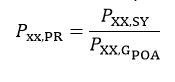# Error Calculation

Given resource and modeling errors input by the user when setting up the simulation parameters, PlantPredict will estimate up to four first year probabilities of exceedance of various annualized metrics. The sources of uncertainty are given in Table 2.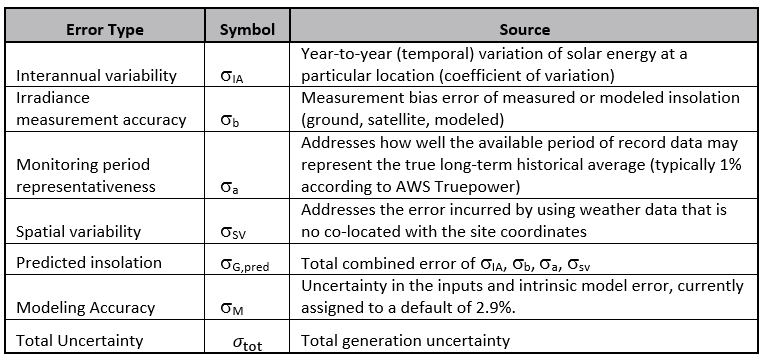### Table 2. Sources of Error

Global horizontal and plane-of-array irradiance uncertainty is computed as follows. For the 1-year values reported in the summary report, the number of years, N, is set to 1: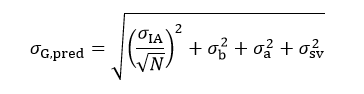The total generated uncertainty affecting DC specific yield, AC capacity factor, Performance Ratio (PR), and total energy, among others, also includes PlantPredict’s internal modeling error: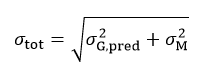The lower confidence interval for all energy metrics, for a desired confidence level (P90, P95) is then computed as follows: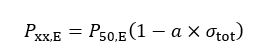Where α is the Z-score for the desired confidence interval and the P50,E is the mean value of the energy metric evaluated. The energy metrics are:

1. Specific Yield
2. AC Capacity Factor
3. Average Array Net Energy
4. Power Plant Net Energy
5. Balance of System (BOS) Losses
6. Non-Netted BOS Station Power

The irradiance metric (GHI and POAI) exceedance probabilities, Pxx,G , are similarly computed, but just applying the irradiance uncertainty to the mean annual irradiance value, P50,G :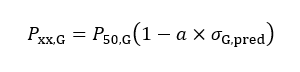The Performance Ratio (PR) is a special case because it is dependent on the plane-of-array irradiance. It is the specific yield at the desired P-level divided by the plane-of-array irradiance at the desired P-level.